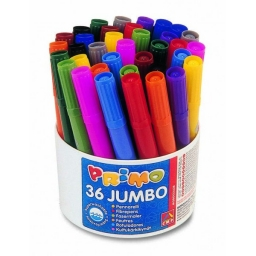# Collection 13863

Vendelín has 4/5 the number of colored pencils. But if Maruška took 1/2 of it, there would be 20 crayons left.

How many crayons were still in the penalty before Vendelín's collection?

p =  50

### Step-by-step explanation:

v = 4/5 p
v - v/2=20

v = 4/5·p
v - v/2=20

4p-5v = 0
v = 40

v = 40/1 = 40
p = 0+5v/4 = 0+5 · 40/4 = 50

p = 50
v = 40

Our linear equations calculator calculates it.Did you find an error or inaccuracy? Feel free to write us. Thank you!

Tips for related online calculators
Need help calculating sum, simplifying, or multiplying fractions? Try our fraction calculator.
Do you have a system of equations and looking for calculator system of linear equations?